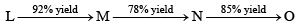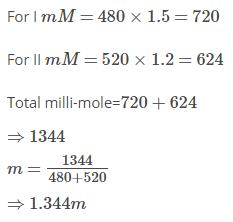Courses

# Test: Mole Concept, Volumetric & Redox - 3

## 30 Questions MCQ Test Physical Chemistry | Test: Mole Concept, Volumetric & Redox - 3

Description
This mock test of Test: Mole Concept, Volumetric & Redox - 3 for Chemistry helps you for every Chemistry entrance exam. This contains 30 Multiple Choice Questions for Chemistry Test: Mole Concept, Volumetric & Redox - 3 (mcq) to study with solutions a complete question bank. The solved questions answers in this Test: Mole Concept, Volumetric & Redox - 3 quiz give you a good mix of easy questions and tough questions. Chemistry students definitely take this Test: Mole Concept, Volumetric & Redox - 3 exercise for a better result in the exam. You can find other Test: Mole Concept, Volumetric & Redox - 3 extra questions, long questions & short questions for Chemistry on EduRev as well by searching above.
QUESTION: 1

Solution:
QUESTION: 2

Solution:
QUESTION: 3

### When the same amount of zinc is treated separately with excess of sulphuric acid and excess of sodium hydroxide, the ratio of volumes of hydrogen evolved is

Solution:
QUESTION: 4

2.76 g of silver carbonate on being strongly heated yields a residue weighing

Solution:
QUESTION: 5

If 0.5 mol of BaCl2 is mixed with 0.20 mol of Na3PO4, the maximum amount of Ba3(PO4)2 that can be formed is

Solution:
QUESTION: 6

A molal solution is one that contains one mole of solute in

Solution:
QUESTION: 7

Which of the following changes with increase in temperature:

Solution:

Temperature will affect the volume of solution.

Hence, the expression for concentration that contain volume will change with temperature. Hence weight fraction of solute changes.

i.e. , Molarity gets effected with the change in temperature because the number of moles per unit volume changes by the change in temperature.

QUESTION: 8

How many moles of electron weighs one kilogram?

Solution:
QUESTION: 9

Which has maximum number of atoms?

Solution:
QUESTION: 10

Given that the abundances of isotopes 54Fe, 56Fe and 57Fe are 5%, 90% and 5% respectively, the atomic mass of Fe is

Solution:
QUESTION: 11

Dissolving 120 g of urea (mol. Wt. 60) in 1000 g of water gave a solution of density 1.15 g/mL. The molarity of the solution is

Solution:
QUESTION: 12

The hydrated salt Na2CO3.x H2O undergoes 63% loss in mass on heating and becomes anhydrous. The value of x is

Solution:
QUESTION: 13

The volume of chlorine at STP required to liberate all the bromine and iodine in 100 mL of 0.1 M each of KI and MBr2 will be:

Solution:
QUESTION: 14

0.5400 g of a metal X yields 1.020 g of its oxide X2O3. The number of moles of X is:

Solution:
QUESTION: 15

Consider the reaction 2A + B + 3C → P + 2Q. Starting with 3 mol of A, 2 mol of B and 6 mol of C, number of moles of the products P and Q would respectively be:

Solution:
QUESTION: 16

A mixture of methane and ethane in the mole ratio of x : y has a mean molecular mass equal to 20. What would be the mean molecular mass of a mixture of the same gases present in the ratio y : x?

Solution:
QUESTION: 17

A partially dried clay mineral contains 8% water. The original sample contained 12% water  and 45% silica. The percentage of silica in the partially dried sample is nearly

Solution:
QUESTION: 18

H2O2 in aqueous solution decomposes on warming as: 2H2O2(aq) → 2H2O(l) + O2(g) If 1 mole of gas occupies a volume of 25 L under the conditions of measurement and 200 ml of x M solution of H2O2 produces 5L of O2, the value of x is

Solution:
QUESTION: 19

5.0 g of a certain element X forms 10.0 g of its oxide having the formula X4O6. The atomic mass of X is

Solution:
QUESTION: 20

Charcoal (1 g) of surface area 100 m2 per gram, adsorbs 60 mg of acetic acid from an aqueous solution at 25°C and 1 atmosphere pressure. The number of moles of acetic acid adsorbed per cm2 of charcoal surface is:

Solution:
QUESTION: 21

The ionic strength of 0.1 M aqueous solution of Fe2(SO4)3 is:

Solution:
QUESTION: 22

In the following sequence of reactions, the overall yield (%) of O is:Solution:
QUESTION: 23

Number of atoms in 558.5 g Fe (at. wt. 55.85) is:

Solution:
QUESTION: 24

In a compound C, H, N atoms are present in 9 : 1 : 3.5 by weight. Molecular weight of compound is 108. Its molecular formula is:

Solution:
QUESTION: 25

What volume of hydrogen gas at 273 K and 1 atm pressure will be consumed in obtaining 21.6 g of elemental boron (at. mass = 10.8) from the reduction of boron trichloride with hydrogen:

Solution:
QUESTION: 26

25 mL of a solution of barium hydroxide on titration with 0.1 molar solution of hydrochloric acid gave a titre value of 35 mL. The molarity of barium hydroxide is:

Solution:
QUESTION: 27

6.02 × 1020 molecules of urea are present in 100 mL solution. The concentration of urea solution is:

Solution:
QUESTION: 28

To neutralize completely 20 mL of 0.1 M aqueous solution of phosphorus (H3PO3) acid the volume of 0.1 M aqueous KOH solution required is:

Solution:
QUESTION: 29

Two solution of a substance (non-electrolyte) are mixed in the following manner,480ml of 1.5M of first solution with 520 ml of 1.2m of second solution.The molarity of final solution is

Solution:QUESTION: 30

If 1/6, in place of 1/12, mass of carbon atom is taken to be the relative atomic mass unit, the mass of one mole of a substance will:

Solution: